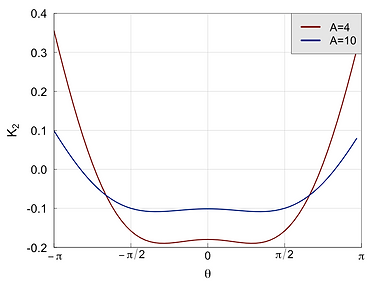top of page

# Compare two tokamak configurations with different aspect ratios: A=10 vs. A=4. Same Itor, different iota.# R=5 m, a=0.5 m# ITG instability with adiabatic electrons: s=0.5, a/LT=2, a/Ln=0.Reducing A leads to more curvature, both good and bad. Increasing the curvature suppresses small scales, but exacerbates the large scales. Based on this picture, we expect stronger turbulence for A=4.# Linear zonal response: s=0.5, Lx=50ρ.Reducing A (iota) leads to smaller ZF residual and less efficient control of turbulence. Based on this picture, we expect stronger turbulence for A=4.# ITG turbulence with adiabatic electrons: s=0.5, a/LT=2, a/Ln=0.The A=4 tokamak has stronger ion heat flux than the A=10 tokamak, according to the expectations above.# Compare W7-X ref=026 (high mirror) vs. W7-K [Roberg-Clark, Plunk and Xanthopoulos, submitted]# W7-K# ITG instability with adiabatic electrons: s=0.5, a/LT=3, a/Ln=0. W7-K increases curvature, both good and bad. Larger scales are exacerbated, smaller scales are suppressed. Suggesting stronger turbulence for W7-K.# Linear ZF response: s=0.5, Lx=25ρ. For W7-K, reducing iota produces weaker ZF response and less effective turbulence control. Suggesting stronger ITG turbulence for W7-K.# ΙΤG turbulence simulations with adiabatic electrons: s=0.5, a/LT=3, a/Ln=0.Turbulence is reduced for W7-K. Evidently, nonlinear effects are involved, not captured by the above analysis.# ITG turbulence is less localized in W7-K than W7-X. This is seen by the distribution of the perturbed density along the tube, based on the "spreading coefficient"                               [Plunk, Xanthopoulos, and Helander, PRL 2017] which is smaller in W7-K. Less localized turbulence is associated with stronger nonlinear ZF drive. (in progress).# The spreading of turbulence is attributed to the flux expansion, geometrically expressed viaFor W7-K the metric is more evenly distributed compared to W7-X.# In W7-K, the flux expansion is not coupled with the elongation. W7-K is, in fact, more elongated than W7-X (perhaps the reason for smaller eps_eff).# The W7-K configuration was conceived to improve the linear ITG threshold for W7-X. However, above marginality, the linear physics is not favorable for W7-K: the growth rates are larger (boosted curvature) and the GAM damping is stronger (small iota). A major surprise, however, occurs in the nonlinear picture, where the ITG turbulence scaling implies both higher nonlinear threshold (left figure) and reduced stiffness (right figure) for W7-K compared to KJM.# The reason for the improved nonlinear performance of W7-K lies in the nonlinear ZF generation. First, to identify the ZF as the key mechanism, we compare ITG turbulence between KJM and W7-K, by artificially removing the ZF response in the simulations. In the figure below we see that, once the ZF is suppressed, the turbulence for W7-K is much stronger (a/LTi=3).# Where does the strong ZF come from for W7-K? The starting point is that the turbulence in W7-K is less localized compared to that for W7-K. This is reflected by a larger "space-filling factor" σ ≃ 0.65 for W7-K compared to σ ≃ 0.40 for W7-X (the smaller the σ, the more localized are the turbulence fluctuations). The σ enters into the nonlinear ZF generation, therefore the ZF drive for W7-K is stronger.# To evaluate this hypothesis, we are working on a model M(γ, ky, σ) which incorporates the space-filling factor σ, in order to reproduce the ion heat flux Q derived directly from GENE simulations. The model is non-predictive, in that it is based on information extracted from the nonlinear simulation. Key quantities are the outer scale ky and |φ| along the tube, both stemming from the nonlinear simulation. Also needed is the growth rate associated with the outer scale. Such model should be applicable if ZF is the main saturation mechanism for the turbulence. In the figure below (left), we show the comparison between the heat flux from GENE and the model for KJM and W7-K. However, once ZF is numerically removed from a simulation, the heat flux resulting from GENE deviates wildly from the model, as expected.# Since the model is not geometry-specific, the question arises if it would hold for any type of configuration, particularly those that rely on ZF for ITG turbulence saturation. A straightforward candidate is the tokamak, which fits nicely with the model.bottom of page